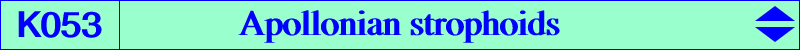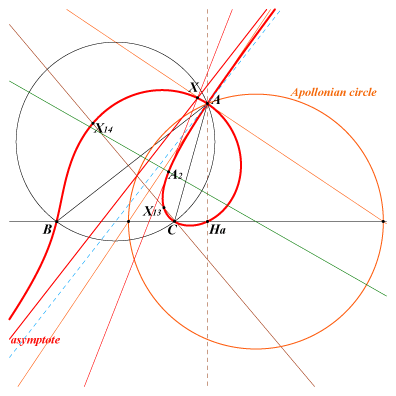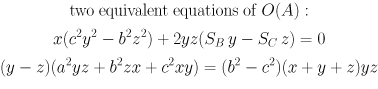X(13), X(14) A2 = A-vertex of second Brocard triangle (singular focus) foot Ha of A-altitude point at infinity of median AG anti-points, see Table 77We meet these three curves in "Orthocorrespondence and Orthopivotal Cubics" §6.5.1. See the FG paper "Orthocorrespondence and orthopivotal cubics" in the Downloads page and Orthopivotal cubics in the glossary. O(A) = K053-A (figure above) is : the orthopivotal cubic with orthopivot A, the isogonal transform of the A-Apollonian circle, the locus of foci of conics tangent at B to AB and at C to AC, the pedal curve with respect to A of the parabola with directrix the median AG, with focus the second intersection of the circumcircle and the A-Apollonian circle or equivalently the A-vertex of the circumcevian triangle of X(6). the locus of P whose antipedal triangle PaPbPc is isosceles at Pa. the locus of M such that the line AM is a bisector of (MB, MC). O(B) = K053-B and O(C) = K053-C have analogous properties obtained by cyclic permutations. These three strophoids generate the net formed by all the orthopivotal cubics and, in particular, the Neuberg cubic, the Brocard (second) cubic, Kn. The isotomic conjugation transforms O(A), O(B), O(C) into three concentric hyperbolas passing through X(298) and X(299), with center X(325) = isotomic conjugate of the Tarry point X(98). See also Paul Yiu's paper "Conic Construction of a Triangle from the Feet of Its Angle Bisectors" here.